Function Repository Resource:

# NegativePedalCurve

Compute the negative pedal curve

Contributed by: Wolfram Staff (original content by Eric W. Weisstein)
 ResourceFunction["NegativePedalCurve"][{a,b},c,t] computes the negative pedal curve with respect to the point {a,b} of the curve c in parameter t.

## Details and Options

The negative pedal of a curve with respect to the point {a,b} is the curve whose pedal is obtained by the reverse of the procedure that generates the pedal curve.
The pedal of a given curve c from a fixed point p is the locus of the foot of the perpendicular from p to the tangent to c.

## Examples

### Basic Examples (2)

Define a cardioid:

 In:=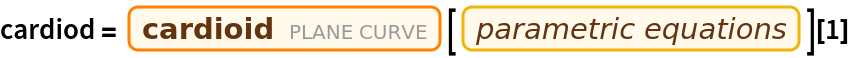Out=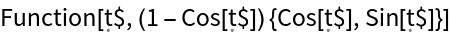Plot the cardioid along with its negative pedal curve:

 In:=Out=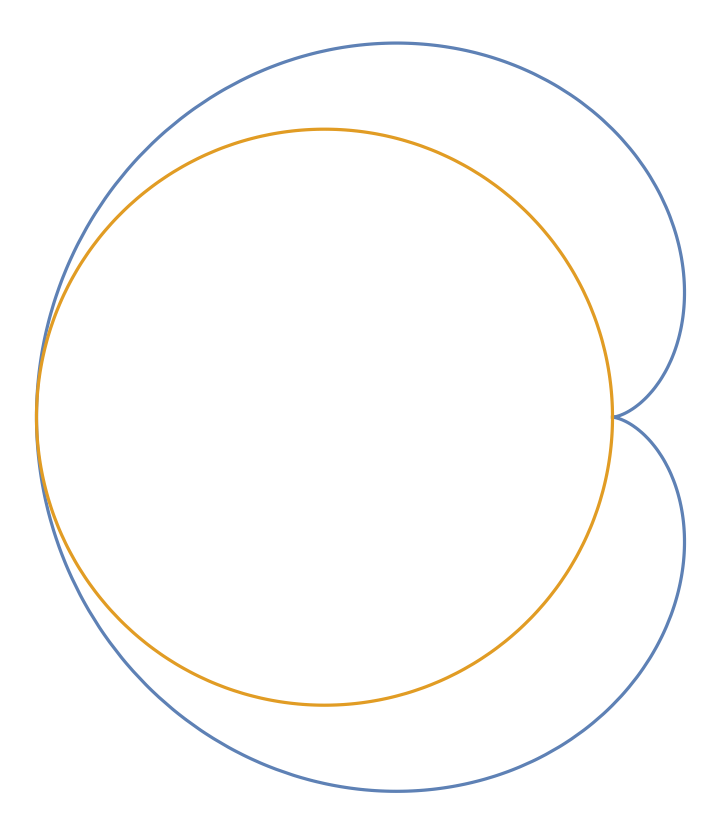### Scope (2)

Define an ellipse:

 In:=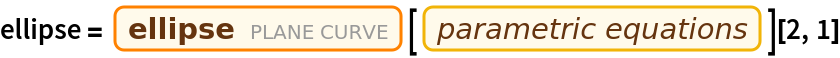Out=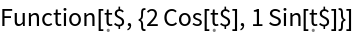Interactively plot the ellipse along with its negative pedal curve, varying the base point:

 In:=Out=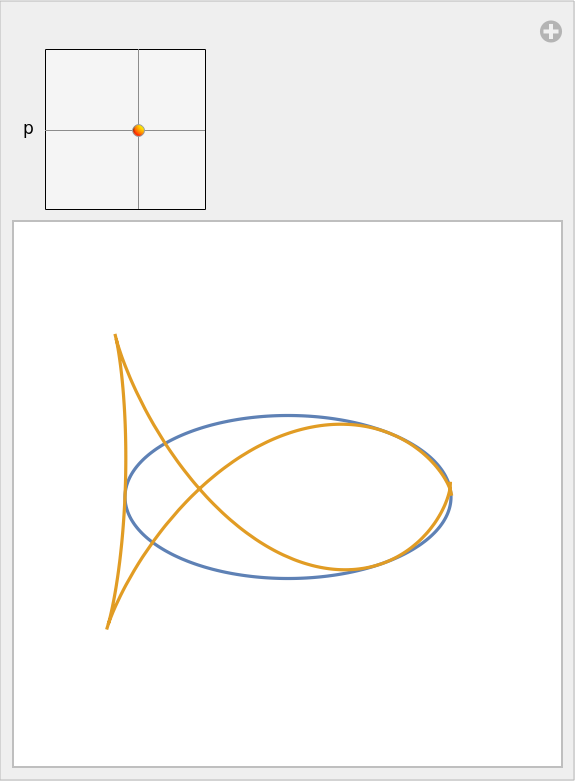Enrique Zeleny

## Version History

• 1.0.0 – 26 February 2020